# Vector Space Model

All NLP applications require a basic understanding of how word vectors are represented in natural language processing. Because the first step in creating any NLP model is to express the word as some kind of number or coding. For instance, a word can be represented as a number or a vector. Additionally, this blog will teach us how to compare several word vectors using two distinct approaches. Known as cosine similarity and Euclidean distance

# Why vector space model used?

Let’s say we have two inquiries.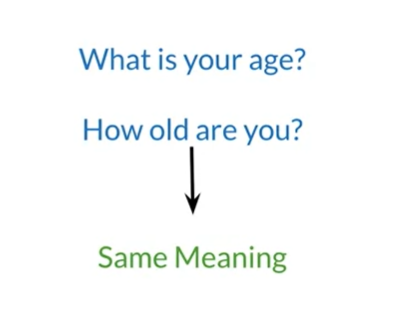All of the words in these sentences—all save the final ones—are the same. They both mean something different, though. On the other hand, suppose we have two additional inquiries, each of which has a distinct set of words but the same overall meaning.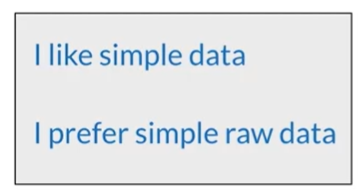Even if the first and second sets of questions do not contain the same exact phrases, vector space models will enable us to determine whether they are similar in meaning. They can be used to spot similarities when summarizing, paraphrasing, and answering questions. Additionally, vector space models will enable us to detect word relationships.

Think at the following sentence

You eat cereal out of a bowl. As you can see, the words cereal and bowl are connected. Let’s examine the second clause: “You buy something, and someone else sells it.” It therefore implies that something is sold because a buyer purchases it. The first half of the statement affects the second part. This and many other kinds of correlations between various collections of words can be captured using vector-based models.

Information extraction uses vector space models to provide answers to questions like who, what, where, how, etc. In sports programming for chess as well as machine translation. They also have a huge variety of other applications. We shall know a word by the company it keeps, according to renowned English linguist John Firth, is a quotation I’d like to leave you with. One of the most essential ideas in NLP is this. The relative meaning of each word in the text is captured by detecting its context when employing vector space models to create representations. Aha! We can represent words and documents as vectors using vector space models. This expresses the underlying meaning.

The use of vector spaces is essential in many NLP applications. A word, paper, tweet, or any other type of text would likely be encoded as a vector if it were to be represented. In processes like information extraction, machine translation, and chatbots, these vectors are crucial. We could also utilize vector spaces to find connections between words.

# How can Construct our Vectors Based on a Co-occurrence Matrix

Different designs may be possible depending on the problem we are trying to solve. We’ll also look at how a word or a page can be encoded as a vector. Let’s demonstrate how we can achieve this. Making a co-occurrence matrix and extracting vector or presentations for the words in our corpus will allow us to create a vector space model using a word-by-word design. Using a similar method, we will be able to create a vector space model word by document. Finally, we’ll demonstrate how we might discover associations between words and vectors in a vector space, often known as their similarity.

## Word By Word Design

The number of times two distinct words appear in our corpus together within a predetermined word distance (k) is known as their co-occurrence. For instance, consider the following two sentences from our corpus,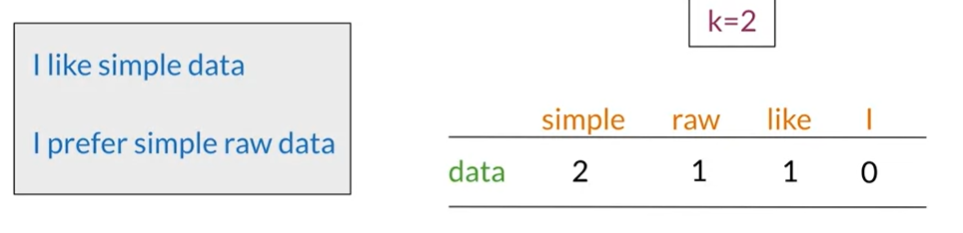The following values would be placed in the roles of the co-occurrence matrices corresponding to the word data with a k value of 2. If we take into account the co-occurrence with the words simple, raw, like, and I, the row of the co-occurrence matrix corresponding to the word data would appear as follows. Data and simple co-occur in the first sentence within a distance of one word and in the second sentence within a distance of two words. The vector representation of the word data in this situation would be 2, 1, 1, 0. We can obtain a representation using a word-by-word design using n.

## Word By Document Design

In this instance, we will tally the frequency with which words from our lexicon appear in texts that fall under particular headings. For instance, we might have a corpus of texts covering a variety of subjects, such as entertainment, the economics, and machine learning. Here, we would need to tally how many times each of the three categories’ documents contained our words.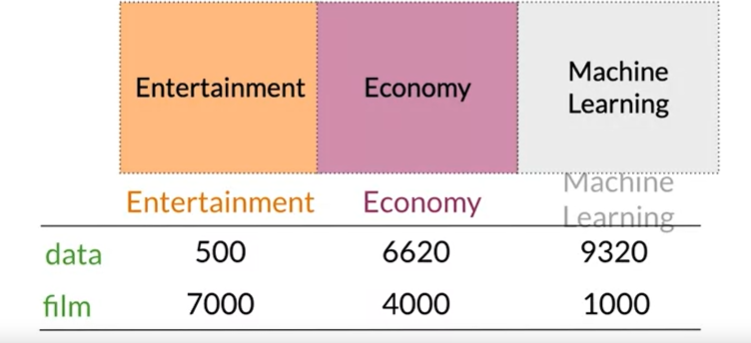Consider the above scenario, In our corpus of documents connected to entertainment, the word data appears 500 times, in documents linked to the economics, 6,620 times, and in documents related to machine learning, 9,320 times. Each document’s category contains the word “film” 7,000, 4,000, and 1,000 times, respectively.

Once we’ve constructed the representations for multiple sets of documents or words, we’ll guess our vector space. Let’s take the matrix from the last example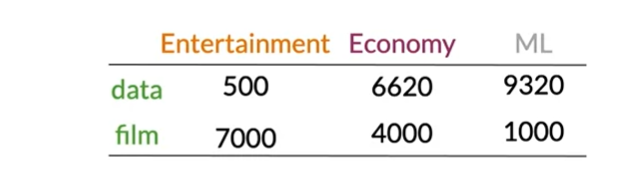From the table’s rows, we may create a representation of the text, information, and movie. However, by examining the columns, we’ll determine how each kind of papers is represented. There will be two dimensions to the vector space. the frequency with which certain words, numbers, or videos appear on a given sort of material. We would have the following vector representations for the entertainment corpus: This one falls under the economics category and pertains to machine learning. Be aware that the economy and machine learning publications in this area are significantly more comparable than they are to the entertainment category.

In order to determine the angle and distance between two vector representations, we will compare them using the cosine similarity and Euclidean distance. So far, we’ve seen two alternative methods for obtaining vector spaces: word-by-word and document-by-word; either by counting the co-occurrence of words or by counting the co-occurrence of words in the documents corpus.

# Euclidean Distance

Euclidean distance, a measure of similarity. We may determine the separation between two points or two vectors using this measure. This section will show you how to calculate the Euclidean distance between two document vectors  and then apply  concept to vector spaces in higher dimensions.

Use two of the corporate vectors we previously employed. Keep in mind that the example used two dimensions. the frequency with which the words “data” and “film” appeared in the corpus. The entertainment corpus will be Corpus A, and the machine-learning corpus will be Corpus B. Let’s now represent those vectors in the vector space as points. The length of the segments of a straight line that connect them is the Euclidean distance. The formula below should be used to determine that value.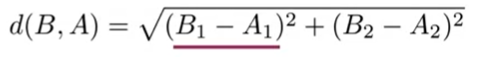Their horizontal distance squared makes up the first phrase, while their vertical distance squared makes up the second. As we can see, the Pythagorean theorem is illustrated by this formula. We should arrive at this expression if we solve for each term in the equation.

The Euclidean distance is not all that much more challenging when we have higher dimensions. Let’s use the ensuing co-occurrence matrix to move through an example.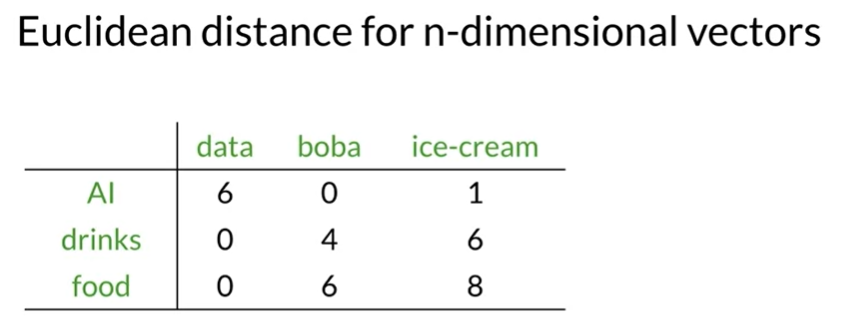Let’s say we wish to calculate the Euclidean distance between the vectors v and w that represent the words ice cream and boba, respectively. We must first determine how different each of their dimensions is. After squaring the discrepancies, adding them all up, we may obtain the square roots of our findings. This equation is known as the norm of the difference between the vectors that we are comparing, if we recall from algebra.

# Cosine Similarity

Cosine Similarity is another types of similarity function. It basically makes use of the cosine of the angle between two vectors. Based on that, it tells us whether two vectors are close or not. We’ll examine the drawbacks of utilizing Euclidean distance, particularly when contrasting vector representations of documents or corpora, and how the cosine similarity measure might help us fix them. Let’s look at the next case to see how the Euclidean distance could be problematic.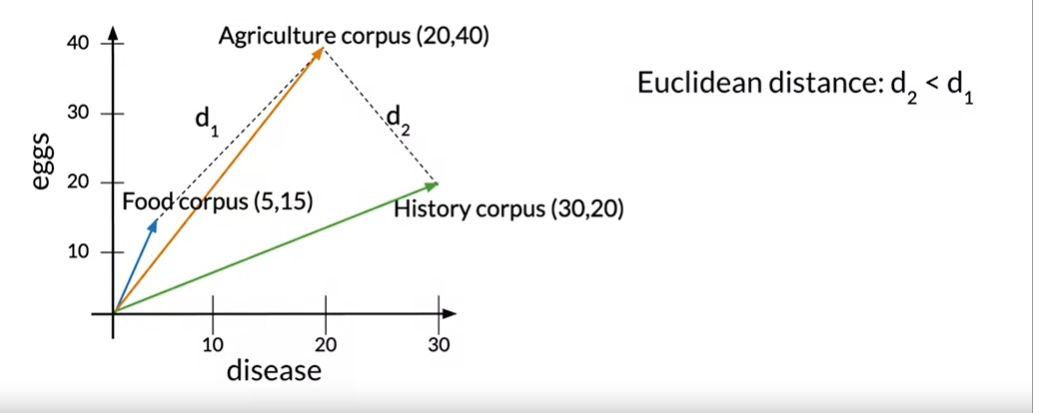A food corpus, an agriculture corpora, and a history corpus are represented here. There are texts on that topic in each of these corpora. However, we are aware that the word totals across the corpora vary. In actuality, the food corpus has comparatively few terms compared to the agriculture and history corpora. Let’s use d 1 and d 2 to denote the Euclidean distances between the food and agriculture corpora and the agriculture and history corpora, respectively. As we can see, the gap between the agricultural and history corpora is smaller than the gap between the agriculture and food corpora, suggesting that the former are more similar than the latter.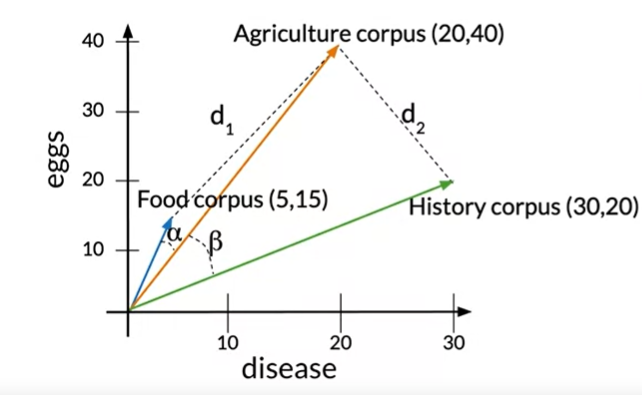Calculating the cosine of two vectors’ inner angles is another typical technique for assessing how similar two vectors are. The cosine would be very near to one if the angle were modest. The cosine moves closer to zero as the angle gets closer to 90 degrees. Here we observe that the angle between food and agriculture is smaller than the angle between agriculture and history, which is the angle beta. In this instance, the cosine of those angles serves as a more accurate substitute for the Euclidean distance in determining how similar these vector representations are to one another.

Now that we understand the basic rationale behind using the cosine similarity as a measure to assess the similarity between two vector representations, let’s put it to use. Keep in mind that this metric’s primary benefit over the Euclidean distance is that it is unaffected by the size disparity between the representations. It is not desirable to use the Euclidean distance if we have two papers that are substantially varied in size. Since the angle between the documents was employed, the cosine similarity did not depend on the size of the corpuses.

# How to Compute the Dot Product and How to find out the Norm

## Norm

This is how a vector’s magnitude, or norm, is expressed. It is described as being equal to the square root of the product of its squared parts. The sum of the products of two vectors’ elements in each vector space dimension is known as the dot product.## Dot Product

The sum of the products of two vectors’ elements in each vector space dimension is known as the dot product.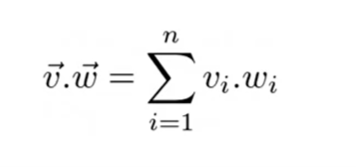## How to find the Angle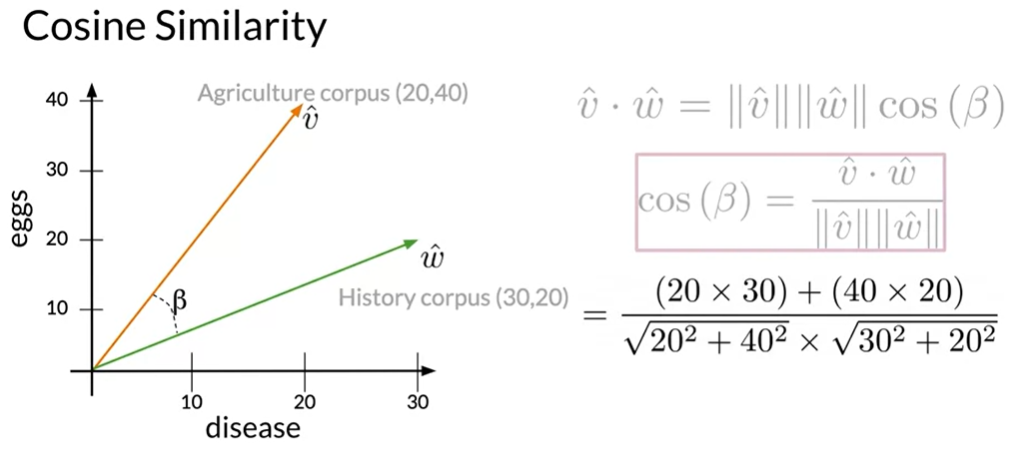Assume we have a vector space in which the representations of the corpora are determined by the frequency with which the phrases sickness and eggs occur. Beta stands for the angle between those vector representations. The history corpus will be represented by vector w, whereas the agriculture corpus is represented by vector v. The following definition applies to the dot products between the vectors. It is clear from this equation that the cosine of the angle Beta is equal to the vectors’ dot product divided by the sum of their two norms. We should get this expression by swapping out the actual values from the vector representations. The product between the instances of the terms sickness and eggs will be in the numerator.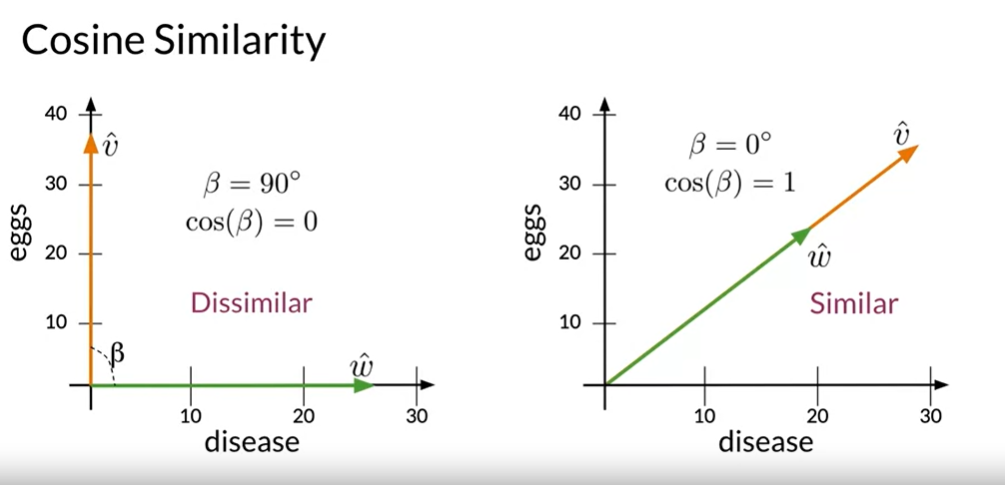What does this metric reveal about the similarity between two distinct vectors, though? In the vector spaces you are familiar with so far, two orthogonal vectors can only have positive values in any dimension. The maximum angle that two vectors can have is 90 degrees. When the cosine is equal to 0, it indicates that the two vectors have orthogonal directions or are as far apart as possible. Let’s now examine the situation where both vectors point in the same direction. In this instance, their angle is 0 degrees, and their cosine value is 1. Cosine of 0 is merely 1, thus.

As we can see, the closer two vectors’ directions are, the closer their cosine of the angle is to 1. Since then, we have learned how to calculate the cosine similarity between any two vectors. It’s important to note that for the vector spaces we’ve examined so far, the cosine similarity takes values between 0 and 1. This metric is proportional to the similarity between the directions of the vectors that we are comparing. We have learned how to calculate the cosine similarity score between two vectors, to sum together. The score is restricted to the range of 0 and 1 for the positive vectors we have observed so far. Be aware that if we compare any vector’s cosine similarity score to itself, we will receive 1. If the vectors are perpendicular, it’ll give you 0. Similar vectors have higher scores.

Tags:

Categories:

Updated: